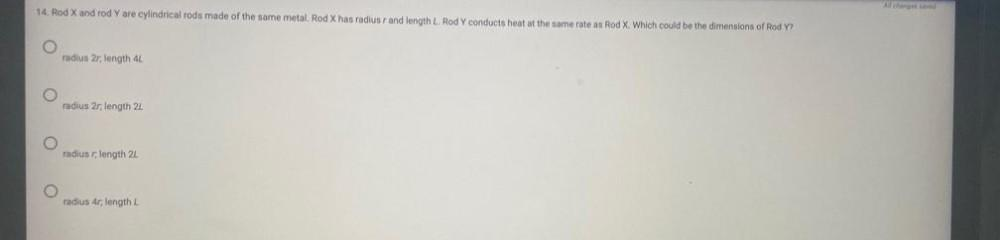Question:

# 14. Rod X and rod Yare cylindrical rods made of the same metal, Rod X has radiusr and length L. Rod Y conducts heat at the same14. Rod X and rod Yare cylindrical rods made of the same metal, Rod X has radiusr and length L. Rod Y conducts heat at the same rate as Rod X Which could be the dimensions of Rod Y? radius 2r; length 44 radius 2r, length 21 radius r, length 21 radius 4r; length